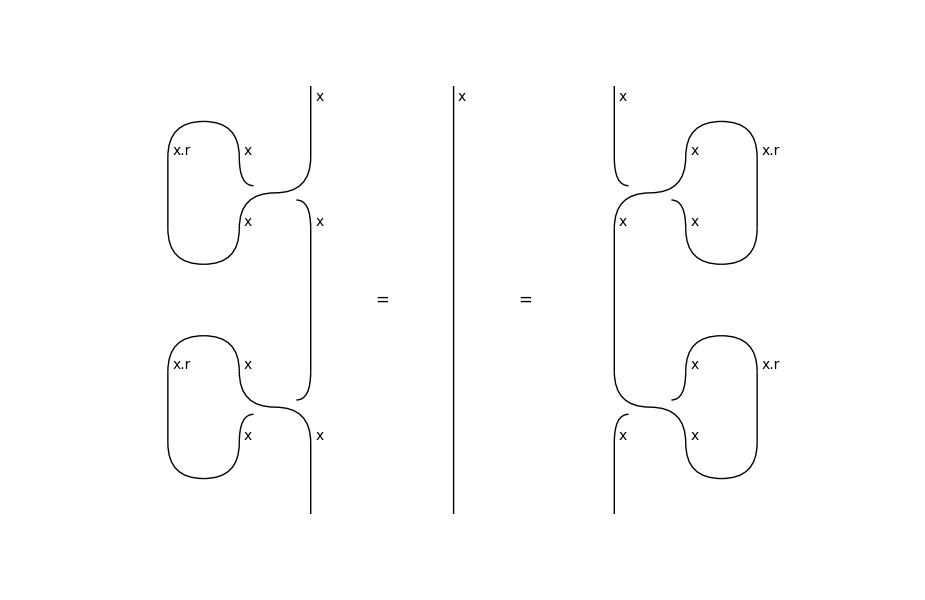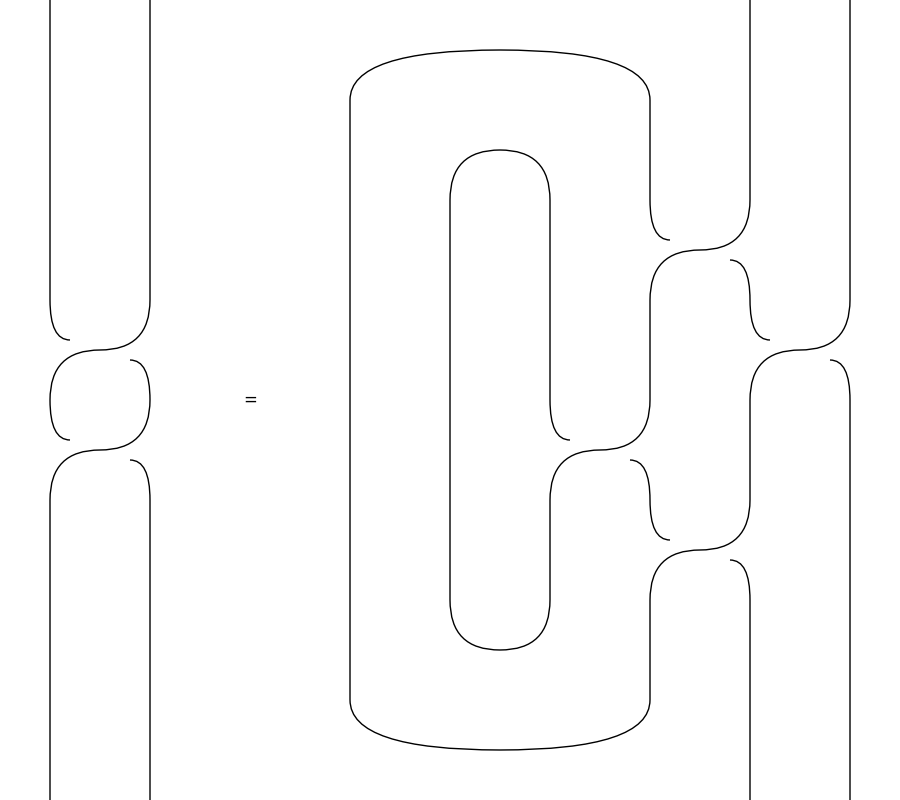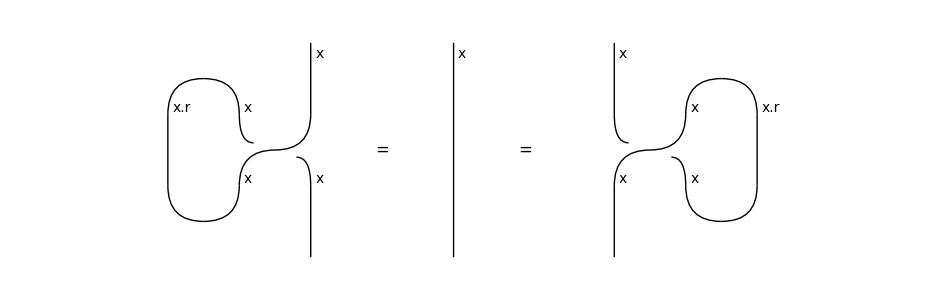# ribbon#

The free ribbon category, i.e. diagrams with braids, cups and caps.

## Summary#

 `Diagram` A ribbon diagram is a pivotal diagram and a balanced diagram. `Box` A ribbon box is a pivotal and balanced box in a ribbon diagram. `Cup` A ribbon cup is a pivotal cup in a ribbon diagram. `Cap` A ribbon cap is a pivotal cap in a ribbon diagram. `Braid` A ribbon braid is a balanced braid in a ribbon diagram. `Category` A ribbon category is both a pivotal category and a balanced category. `Functor` A ribbon functor is both a pivotal functor and a balanced functor.

## Axioms#

A ribbon category is a braided pivotal category, such that the trace of the braid is unitary.

```>>> x = Ty('x')
>>> from discopy.drawing import Equation
>>> twist_l = Braid(x, x).trace(left=True)
>>> twist_r = Braid(x, x).trace(left=False)
>>> eq = Equation(twist_l >> twist_l[::-1], Id(x), twist_r >> twist_r[::-1])
>>> eq.draw(figsize=(6, 4), margins=(.2, .1),
...         path='docs/_static/ribbon/twist-untwist.png')
```Equivalently, a ribbon category is a balanced pivotal category, such that the twist is the trace of the braid [Sel10]. This is made explicit by drawing wires as ribbons, i.e. two parallel wires with the twist drawn as the double braid.

```>>> ribbon_twist = Diagram.twist(x).to_ribbons()
>>> eq = Equation(ribbon_twist, twist_l.to_ribbons())
>>> eq.draw(symbol='\$\\mapsto\$', draw_type_labels=False,
...     path="docs/_static/balanced/ribbon_twist.png")
```A ribbon category is strict whenever the twist is the identity. Strict ribbon categories have diagrams with knots, i.e. ribbons where the two parallel wires coincide and the twist is the identity.

```>>> eq_strict = Equation(twist_l, Id(x), twist_r)
>>> eq_strict.draw(figsize=(4, 2), margins=(.2, .1),
...                path='docs/_static/ribbon/strict.png')
```